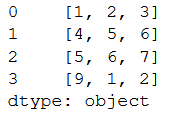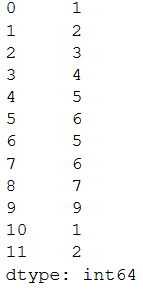# Converting Series of lists to one Series in Pandas

Let us see how to connvert a Series of lists to a single Series in Pandas.

First of all let us create a Series of lists. For this problem we have created a Series of list having 12 elements, each list is of length 3 and there are 4 lists hence total elements are 12. When the Series of list will be converted to one it will have 12 elements.

## Python3

 `import` `pandas as pd ` `data ``=` `[[``1``, ``2``, ``3``], [``4``, ``5``, ``6``], [``5``, ``6``, ``7``], [``9``, ``1``, ``2``]] ` ` `  `s ``=` `pd.Series(data) ` `print``(s) `

Output:Method 1: Using the extend() method

In this method, we iterate over each element of the Series and add it to a list, note that we are using the extend() method.

## Python3

 `ls ``=` `[] ` ` `  `for` `i ``in` `s: ` `    ``ls.extend(i) ` ` `  `s1 ``=` `pd.Series(ls) ` `print``(s1)`

Output:Method 2 : Using pd.concat() method

We iterate through each list in the series and convert it to Series object then we concatenate all the series along the rows to form the final Series. We also need to reset the index

## Python3

 `ls ``=` `[] ` `for` `i ``in` `s: ` `    ``ls.append(pd.Series(i)) ` ` `  `s1 ``=` `pd.concat([``*``ls]).reset_index(drop ``=` `True``) ` ` `  `print``(s1) `

Output:Method 3: Using the apply() method.

The Series object has an apply() method which takes in a function as an argument and applies it to every element. We then stack all the elements and reset the index .

## Python3

 `s1 ``=` `s.``apply``(pd.Series).stack().reset_index(drop ``=` `True``) ` `print``(s1) `

Output:Attention geek! Strengthen your foundations with the Python Programming Foundation Course and learn the basics.

To begin with, your interview preparations Enhance your Data Structures concepts with the Python DS Course.

My Personal Notes arrow_drop_upCheck out this Author's contributed articles.

If you like GeeksforGeeks and would like to contribute, you can also write an article using contribute.geeksforgeeks.org or mail your article to contribute@geeksforgeeks.org. See your article appearing on the GeeksforGeeks main page and help other Geeks.

Please Improve this article if you find anything incorrect by clicking on the "Improve Article" button below.

Article Tags :

Be the First to upvote.

Please write to us at contribute@geeksforgeeks.org to report any issue with the above content.